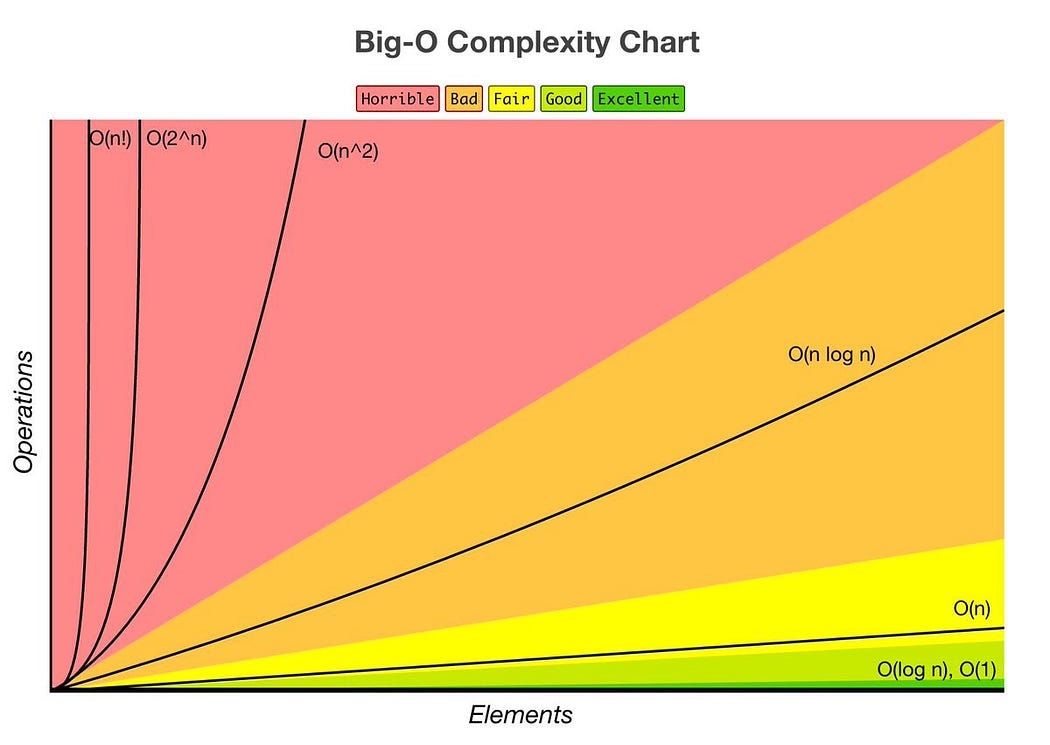# Lecture 1: Algorithms and Computation

## Algorithms and Computation

The value of learning about algorithms are:

• Solving computational problems
• Proving correctness
• Argue the efficiency of your algorithm
• Communicate your ideas to others

### Solving computational problems

A computational problem is a binary relation between the problem inputs and the correct outputs. An example of a computational problem is sorting numbers in an array or list.

Solving a problem involves designing an algorithm. An algorithm is a function that accepts arbitrary inputs and maps them to deterministic output(s).

### Proving correctness

A program’s correctness is proven using inductive reasoning.

### Argue efficiency

An algorithm’s efficiency is measured by how fast it produces the correct output. Efficiency is measured by counting the number of operations(ops).

The efficiency of an algorithm is annotated using asymptotic analysis. The annotations used include:

• Big O: measures the upper bound or worst-case scenario
• Omega (Ω): measures the lower bound or best case scenario
• Theta (θ): measures the average case

Example complexity annotations:

• O(1): constant. The computation time will be the same regardless of the input size.
• O(log n): logarithmic.
• O(n): linear. Computation time increases when the data set is doubled.
• O(n logn): log-linear
Illustration of the complexities